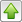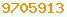# O(n²) : Java Glossary

o(n²)
It is a way of roughly comparing the speed of algorithms. Big O notation is a way of categorising how algorithms will perform as they are scaled up to handle more data. Most algorithms perform acceptably well when the number of items to process is small, but some become impossibly slow as the number of items increases.

If you invented a sorting algorithm that was o(n²) that means the sorting time is proportional to n² where n in the number of elements to sort. An o(n) sort would be one that has constant time per item, proportional to the number of items. An o(n²) algorithm might beat an o(n) algorithm for small n, but above some number, the o(n) will be faster.

Meaning of O(n) Notation
Notation Meaning
O(1) means constant. It does not depend on how many items there are, e.g. indexing an array.
O(n) algorithm is linear. The time it will take is proportional to n. It takes the same amount of time to handle each item no matter how many items there are. RadixSort is O(n).
O(n log(n)) Most of the commonly used sorts.
O(n²) An algorithm varies as the square of the number of times. If you have twice as many items to process it will take four times as long. Most novice sort have this unfortunate property.
There is a subtle difference between O(n²) and o(n²), Big-O vs Landau notation.This page is postedon the web at: http://mindprod.com/jgloss/onsquared.html Optional Replicator mirror of mindprod.com on local hard disk J: J:\mindprod\jgloss\onsquared.htmlPlease read the feedback from other visitors, or send your own feedback about the site.Contact Roedy. Please feel free to link to this page without explicit permission. Canadian Mind Products IP:[65.110.21.43] Your face IP:[44.212.94.18] Feedback You are visitor number